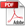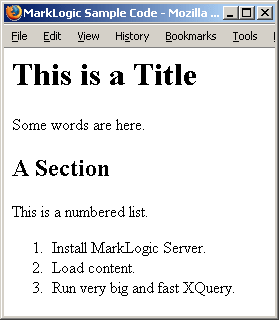# Application Developer's Guide — Chapter 7

## Transforming XML Structures With a Recursive typeswitch Expression

A common task required with XML is to transform one structure to another structure. This chapter describes a design pattern using the XQuery `typeswitch` expression which makes it easy to perform complex XML transformations with good performance, and includes some samples illustrating this design pattern. It includes the following sections:

### XML Transformations

Programmers are often faced with the task of converting one XML structure to another. These transformations can range from very simple element name change transformations to extremely complex transformations that reshape the XML structure and/or combine it with content from other documents or sources. This section describes some aspects of XML transformations and includes the following sections:

#### XQuery vs. XSLT

XSLT is commonly used in transformations, and it works well for many transformations. It does have some drawbacks for certain types of transformations, however, especially if the transformations are part of a larger XQuery application.

XQuery is a powerful programming language, and MarkLogic Server provides very fast access to content, so together they work extremely well for transformations. MarkLogic Server is particularly well suited to transformations that require searches to get the content which needs transforming. For example, you might have a transformation that uses a lexicon lookup to get a value with which to replace the original XML value. Another transformation might need to count the number of authors in a particular collection.

#### Transforming to XHTML or XSL-FO

A common XML transformation is converting documents from some proprietary XML structure to HTML. Since XQuery produces XML, it is fairly easy to write an XQuery program that returns XHTML, which is the XML version of HTML. XHTML is, for the most part, just well-formed HTML with lowercase tag and attribute names. So it is common to write XQuery programs that return XHTML.

Similarly, you can write an XQuery program that returns XSL-FO, which is a common path to build PDF output. Again, XSL-FO is just an XML structure, so it is easy to write XQuery that returns XML in that structure.

#### The typeswitch Expression

There are other ways to perform transformations in XQuery, but the `typeswitch` expression used in a recursive function is a design pattern that is convenient, performs well, and makes it very easy to change and maintain the transformation code.

For the syntax of the `typeswitch` expression, see The typeswitch Expression in XQuery and XSLT Reference Guide. The `case` clause allows you to perform a test on the input to the `typeswitch` and then return something. For transformations, the tests are often what are called kind tests. A kind test tests to see what kind of node something is (for example, an element node with a given QName). If that test returns true, then the code in the `return` clause is executed. The `return` clause can be arbitrary XQuery, and can therefore call a function.

Because XML is an ordered tree structure, you can create a function that recursively walks through an XML node, each time doing some transformation on the node and sending its child nodes back into the function. The result is a convenient mechanism to transform the structure and/or content of an XML node.

### Sample XQuery Transformation Code

This section provides some code examples that use the `typeswitch` expression. For each of these samples, you can cut and paste the code to execute against an App Server. For a more complicated example of this technique, see the Shakespeare Demo Application on developer.marklogic.com/code.

The following samples are included:

#### Simple Example

The following sample code does a trivial transformation of the input node, but it shows the basic design pattern where the `default` clause of the `typeswitch` expression calls a simple function which sends the child nodes back into the original function.

```xquery version "1.0-ml";

(: This is the recursive typeswitch function :)
declare function local:transform(\$nodes as node()*) as node()*
{
for \$n in \$nodes return
typeswitch (\$n)
case text() return \$n
case element (bar) return <barr>{local:transform(\$n/node())}</barr>
case element (baz) return <bazz>{local:transform(\$n/node())}</bazz>
case element (buzz) return
<buzzz>{local:transform(\$n/node())}</buzzz>
case element (foo) return <fooo>{local:transform(\$n/node())}</fooo>
default return <temp>{local:transform(\$n/node())}</temp>
};

let \$x :=
<foo>foo
<bar>bar</bar>
<baz>baz
<buzz>buzz</buzz>
</baz>
foo
</foo>
return
local:transform(\$x)```

This XQuery program returns the following:

```<fooo>
foo
<barr>bar</barr>
<bazz>baz
<buzzz>buzz</buzzz>
</bazz>
foo
</fooo>```

#### Simple Example With cts:highlight

The following sample code is the same as the previous example, except it also runs cts:highlight on the result of the transformation. Using cts:highlight in this way is sometimes useful when displaying the results from a search and then highlighting the terms that match the `cts:query` expression. For details on cts:highlight, see Highlighting Search Term Matches in the Search Developer's Guide.

```xquery version "1.0-ml";

(: This is the recursive typeswitch function :)
declare function local:transform(\$nodes as node()*) as node()*
{
for \$n in \$nodes return
typeswitch (\$n)
case text() return \$n
case element (bar) return <barr>{local:transform(\$n/node())}</barr>
case element (baz) return <bazz>{local:transform(\$n/node())}</bazz>
case element (buzz) return
<buzzz>{local:transform(\$n/node())}</buzzz>
case element (foo) return <fooo>{local:transform(\$n/node())}</fooo>
default return <booo>{local:transform(\$n/node())}</booo>
};

let \$x :=
<foo>foo
<bar>bar</bar>
<baz>baz
<buzz>buzz</buzz>
</baz>
foo
</foo>
return
cts:highlight(local:transform(\$x), cts:word-query("foo"),
<b>{\$cts:text}</b>)```

This XQuery program returns the following:

```<fooo>
<b>foo</b>
<barr>bar</barr>
<bazz>baz
<buzzz>buzz</buzzz>
</bazz>
<b>foo</b>
</fooo>```

#### Sample Transformation to XHTML

The following sample code performs a very simple transformation of an XML structure to XHTML. It uses the same design pattern as the previous example, but this time the XQuery code includes HTML markup.

```xquery version "1.0-ml";
declare default element namespace "http://www.w3.org/1999/xhtml";

(: This is the recursive typeswitch function :)
declare function local:transform(\$nodes as node()*) as node()*
{
for \$n in \$nodes return
typeswitch (\$n)
case text() return \$n
case element (a) return local:transform(\$n/node())
case element (title) return <h1>{local:transform(\$n/node())}</h1>
case element (para) return <p>{local:transform(\$n/node())}</p>
case element (sectionTitle) return
<h2>{local:transform(\$n/node())}</h2>
case element (numbered) return <ol>{local:transform(\$n/node())}</ol>
case element (number) return <li>{local:transform(\$n/node())}</li>
default return <tempnode>{local:transform(\$n/node())}</tempnode>
};

let \$x :=
<a>
<title>This is a Title</title>
<para>Some words are here.</para>
<sectionTitle>A Section</sectionTitle>
<para>This is a numbered list.</para>
<numbered>
<number>Install MarkLogic Server.</number>
<number>Run very big and fast XQuery.</number>
</numbered>
</a>
return
<html xmlns="http://www.w3.org/1999/xhtml">
<body>{local:transform(\$x)}</body>
</html>```

This returns the following XHTML code:

```<html xmlns="http://www.w3.org/1999/xhtml">
<title>MarkLogic Sample Code</title>
<body>
<h1>This is a Title</h1>
<p>Some words are here.</p>
<h2>A Section</h2>
<p>This is a numbered list.</p>
<ol>
<li>Install MarkLogic Server.</li>
<li>Run very big and fast XQuery.</li>
</ol>
</body>
</html>```

If you run this code against an HTTP App Server (for example, copy the code to a file in the App Server root and access the page from a browser), you will see results similar to the following:Note that the `return` clauses of the `typeswitch` `case` statements in this example are simplified, and look like the following:

`case element (sectionTitle) return <h2>{local:passthru(\$x)}</h2>`

In a more typical example, the `return` clause would call a function:

`case element (sectionTitle) return local:myFunction(\$x)`

The function can then perform arbitrarily complex logic. Typically, each case statement calls a function with code appropriate to how that element needs to be transformed.

#### Extending the typeswitch Design Pattern

There are many ways you can extend this design pattern beyond the simple examples above. For example, you can add a second parameter to the simple `transform` functions shown in the previous examples. The second parameter passes some other information about the node you are transforming.

Suppose you want your transformation to exclude certain elements based on the place in the XML hierarchy in which the elements appear. You can then add logic to the function to exclude the passed in elements, as shown in the following code snippet:

```declare function transform(\$nodes as node()*, \$excluded as element()*)
as node()*
{
(: Test whether each node in \$nodes is an excluded element, if so
return empty, otherwise run the typeswitch expression.
:)
for \$n in \$nodes return
if ( some \$node in \$excluded satisfies \$n )
then ( )
else ( typeswitch (\$n) ..... )
};```

There are plenty of other extensions to this design pattern you can use. What you do depends on your application requirements. XQuery is a powerful programming language, and therefore these types of design patterns are very extensible to new requirements.

« Previous chapter
Next chapter »